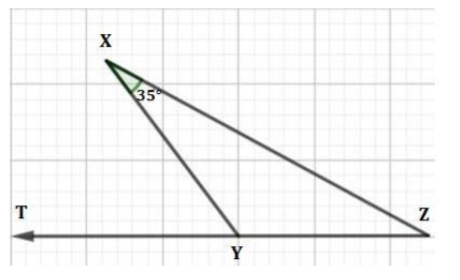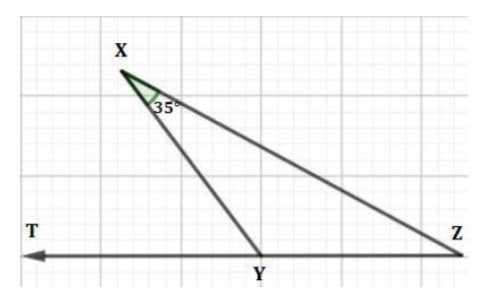QuestionAnswers

# If in the given figure, XY = YZ then, what is the value of $\angle XYT$?(a) 35$^{\circ }$(b) 100$^{\circ }$(c) 110$^{\circ }$(d) 70$^{\circ }$

Hint: We need to know about two properties before solving this question, first is that in an isosceles triangle angles made by the equal sides with the other side of the triangle are equal. And secondly, the sum of linear pair of angles is 180$^{\circ }$ i.e. they are supplementary angles.

Complete step-by-step solution:
We are given that in the below figure,XY = YZ, and
$\angle YXZ$ = 35$^{\circ }$
If XY = YZ then $\Delta XYZ$ is an isosceles triangle and we know that,
In an isosceles triangle angles made by the equal sides with the other side of the triangle are equal, so
$\angle YXZ=\angle YZX$,
Hence we get
$\angle YZX={{35}^{\circ }}$
Now using the property of the triangles that sum of all three interior angles of a triangle is equal to 180$^{\circ }$, we get
$\angle YXZ+\angle YZX+\angle XYZ={{180}^{\circ }}$
Hence, we have
\begin{align} & {{35}^{\circ }}+{{35}^{\circ }}+\angle XYZ={{180}^{\circ }} \\ & \angle XYZ={{180}^{\circ }}-{{70}^{\circ }} \\ & \angle XYZ={{110}^{\circ }} \\ \end{align}
Now, we know that sum of the linear pair of angles is 180$^{\circ }$, and we can observe that$\angle XYZ\,\,and\,\,\angle XYT$ are linear pair of angles hence,
\begin{align} & \angle XYT+\angle XYZ={{180}^{\circ }} \\ & \angle XYT={{180}^{\circ }}-\angle XYZ \\ & \angle XYT={{180}^{\circ }}-{{110}^{\circ }} \\ & \angle XYT={{70}^{\circ }} \\ \end{align}
Hence, we got our answer as 70$^{\circ }$
Our answer matches with option (d) hence, it is the correct answer.

Note: We can also solve this question directly as we know that the exterior angle of a triangle is equal to the sum of two opposite interior angles of the same triangle and we already know that the $\angle YXZ=\angle YZX={{35}^{\circ }}$
Hence,
$\angle XYT=\angle YXZ+\angle YZX={{35}^{\circ }}+{{35}^{\circ }}={{70}^{\circ }}$
So, this is a very easy method through which we can solve our given problem.